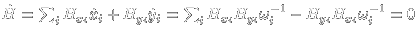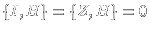Vortex motion

The motion of N vortices with voriticitylocated at points zi in the complex planeis determined by the differential equationsThis is a Hamiltonian systemfor the variables xi+i yi=zi. The Hamiltonian function or energy isUsing the notationone can writethe variablesare canonically conjugated. The phase space of the system is, whereis the collision set.

The N vortex system has 4 classical integrals of motion: two integrals for the center of mass, one integral for the angular momentumand one integral for the energy H. The center of mass is invariant because every term inappears twice with opposite sign. To see the angular momentum invariance, defineBecause I andare real,holds andis purely imaginary so that. The energy is invariant because. These integrals come from symmetry: the translational, rotational and time invariance of the differential equations. With the Poisson bracketfor two smooth functions, the notationand the scalar productonone hasand the equations of motion areorThe invariance of the integrals means. Other integrals are obtained by combining known ones. For exampleis an integral.

Because H,I,J have pairwise non-vanishing Poisson brackets, a 3 vortex system is explicitly integrable. The threshold for chaotic behavior starts with N=4. Already the restricted 4-vortex system is non-integrable. It is the limiting case, where one of the vortices does not contribute to the vorticity.

If the vorticities are positive and, the solution of the vortex flow exists for all times becausedefines a compact set and because H=const prevents collisions.

Iftakes both signs the existence and uniqueness problem is nontrivial and catastrophes are possible: the 3-vortex situationandfor example leads to a collapse at time: if ai are the lengths of the triangle formed by z1,z2,z3and A is the triangle area, one has. The ratios of the triangle lengths are conserved implyingso that.

Kolmogorov-Arnold- Moser theory implies that there exists always quasi-periodic motion in the phase space of a N-vortex system. A vortex system of four or more vortices shows therefore a mixture of stable and unstable behavior.

 Animation Webtechnical information on these pages

 © 2000, Oliver Knill , dynamical-systems.org